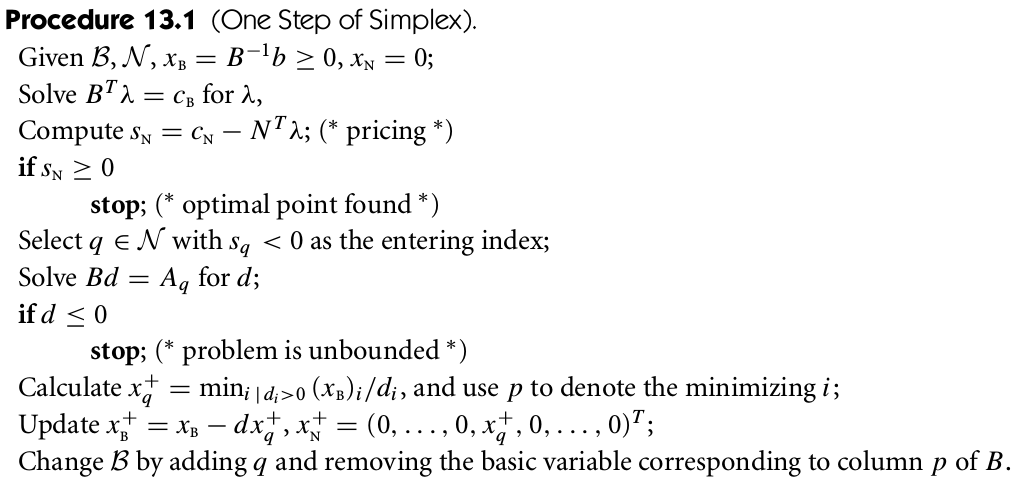# The Simplex Method

##### Posted on Jul 23, 2019
Tags: Linear Programming

This note is based on Chapter 13 of Nocedal, J., & Wright, S. (2006). Numerical optimization. Springer Science & Business Media.

Consider

$\begin{equation} \min\; c^Tx,\qquad \text{subject to }Ax=b,x\ge 0\,,\label{13.1} \end{equation}$

where $c$ and $x$ are vectors in $\IR^n$, $b\in\IR_m$, and $A$ is an $m\times n$ matrix.

The Lagrangian function is

$\cL(x,\lambda,s) = c^Tx-\lambda^T(Ax-b)-s^Tx$

and the KKT condition is

\begin{align*} A^T\lambda + s &=c\\ Ax&=b\\ x&\ge 0\\ s&\ge 0\\ x_is_i&=0,\;i=1,\ldots,n\,. \end{align*}

Each iterate generated by the simplex method is a basic feasible point of \eqref{13.1}. A vector $x$ is a basic feasible point if it is feasible and if there exists a subset $\cB$ of the index set ${1,2,\ldots,n}$ such that

• $\cB$ contains exactly $m$ indices
• $i\not\in\cB\Rightarrow x_i=0$
• The $m\times m$ matrix $B$ defined by $B=[A_i]_{i\in\cB}$ is nonsingular, where $A_i$ is the $i$-th column of $A$.
All basic feasible points for \eqref{13.1} are vertices of the feasible polytope $\{x\mid Ax=b,x\ge 0\}$, and vice versa.

Let $\cN=\{1,2,\ldots,n\}\backslash\cB$ be the nonbasic index set, and partition the $n$-element vectors $x,s$ and $c$ according to the index sets $\cB$ and $\cN$.

By the KKT condition,

$Ax = Bx_B+Nx_N=b\,,$

since we are dealing with basic feasible points, and know that $B$ is nonsingular and that $x_B\ge 0$, then we have

$x_B=B^{-1}b,\qquad x_N=0\,.$

Choose $s$ to satisfy the complementarity condition by setting $s_B=0$, then

$B^T\lambda = c_B,\qquad N^T\lambda+s_N=c_N\,,$

it follows that

$\lambda = B^{-T}c_B$

and

$s_N = c_N-N^T\lambda = c_N-(B^{-1}N)^Tc_B\,.$

The only KKT condition that not enforced explicitly is the nonnegativity conditions $s\ge 0$.

• If $s_N\ge 0$, terminate and declare success
• Choose onr of the indices $q\in\cN$ for which $s_q < 0$ to be the entering index.

The procedure for altering $\cB$ and changing $x$ and $s$ is as follows:

• allow $x_q$ to increase from zero during the next step
• fix all other components of $x_N$ at zero, and figure out the effect of increasing $x_q$ on the current basic vector $x_B$, given that staying feasible w.r.t. the equality constraints $Ax=b$.
• keep increasing $x_q$ until one of the components of $x_B$ ($x_p$, say) is driven to zero, or determining that no such component exists (the unbounded case)
• remove the leaving index $p$ from $\cB$ and replace it with the entering index $q$.

Since both the new iterate $x^+$ and the current iterate $x$ should satisfy $Ax=b$, and since $x_N=0$ and $x_i^+=0$ for $i\in\cN\backslash\{q\}$, we have

$Ax^+=Bx_B^++A_qx_q^+=Bx_B=Ax\,,$

thus

$x_B^+=x_B-B^{-1}A_qx_q^+\,,$

which is usually a move along an edge of the feasible polytope that decrease $c^Tx$, and we continue to move along this edge until a new vertex is encountered.

Note that

\begin{align*} c^Tx^+=c_B^Tx_B^++c_qx_q^+&=c_B^Tx_B-c_B^TB^{-1}A_qx_q^++c_qx_q^+\\ &=c_B^Tx_B-\lambda^TA_qx_q^++c_qx_q^+\\ &=c_B^Tx_B-(c_q-s_q)x_q^++c_qx_q^+\\ &=c^Tx+s_qx_q^+\,. \end{align*}

Since $q$ was chosen to have $s_q < 0$, then the above step produces a decrease in the primal objective function $c^Tx$ whenever $x_q^+>0$.

It is possible that we can increase $x_q^+$ to $\infty$ without ever encountering a new vertex. When this happens, the linear program is unbounded.Published in categories Note